×

Search anything:

# 2D Sliding Window

#### Algorithms Sliding Window techniqueIn this article, we have explored how to apply the concept of Sliding Window on a 2D array that is 2D Sliding Window. We have presented the concept along with implementation and time and space complexity.

• Sliding Window
• Use of Sliding Window
• Sliding window on a 1D array
• Sliding window on a 2D array
• Time and Space complexity of 2D sliding window

# Sliding Window

Several methods are used for solving computational problems. These include arithmetic operations, loops, conditional statements, and their usage for complex problems is made easy through the application of algorithms.

An algorithm is a set of rules that solves a complicated problem. It takes input and processes it step-by-step using the basic techniques to easily accomplish a task. Types of algorithms include the Brute Force algorithm, Greedy algorithm, Backtracking algorithm, and several others.

Such an algorithm is Sliding Window and it is used on problems involving arrays or lists. This technique is used for making calculations easy that include nested loops. The concept of this algorithm is as it sounds. It selects a specific length of an array or list for computational purposes and then slides through that list picking different portions of that same length. The process is explained with an example: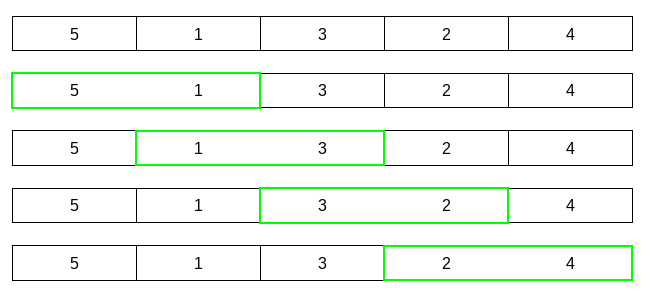This is a list of numbers. A k = 2 window will move through this list by taking two items at a time until it reaches the end of the list. The window is highlighted in green color.

# Use of Sliding Window

The sliding window technique can be used in limited circumstances. It can only be applied if the window size stays fixed throughout the process. It tends to make programs run faster by replacing nested loops with a single loop.

The steps of this algorithm are:

1. Fixed window size for choosing elements
For example, window size = 1 & array = {8,3,5}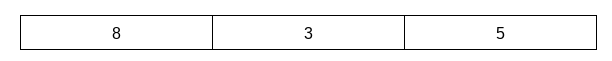2. Computation of that window from the start of a list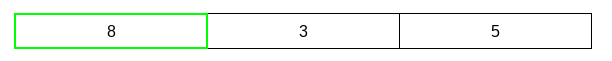3. Sliding that window by 1 and continue computing the result until the end of that list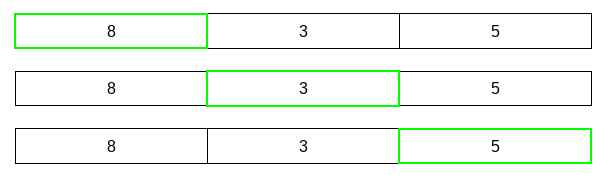The applications of the sliding window:

• Calculating average
• Finding minimum/maximum value
• Finding the longest/shortest value
• Applied on an ordered data structure
• Finding a target element from a list/array
• Finding selected sized pair of elements

# Sliding window on a 1D array

In the case of a 1D array, there are no columns. It only has a row and A has indices:
[ 0, 1, 2, 3, 4]

To find the maximum sum of length two from this array, we take two elements from the start and compute the sum. Then we move one index to the right to select two items for summation and compare the results. The process is repeated until the maximum sum is found.

To calculate this in code, we will need a loop for iterating through the array and inside that loop will be another loop to select items of a specific length.
Application of sliding window to find the maximum sum of two items from the array
A = { 2, 1, 4, 3, 0 }: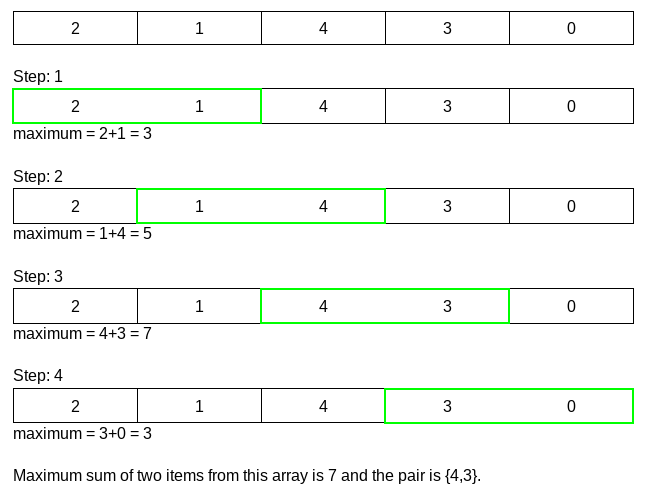Maximum sum of two items from this array is 7 and the pair is {4,3}.
Using the same method, we can find the maximum/minimum value from the array, and find a specific item.

# Sliding window on a 2D array

2D arrays are used for representing matrices. An array of arrays indicates the rows and columns of a matrix. They have specific indices indicating their location in the array. The elements of the 2D array can be accessed using the indices of the rows and columns.

A 2d array A has indices:
[ {0,0}, {0,1}, {0,2},
{1,0}, {1,1}, {1,2},
{2,0}, {2,1}, {2,2} ]

So, to find a value only the index of the row and the index of the column are needed. Nested loops are used to present a 2D array in code. One loop is for the rows and the other is for the columns.

Applying 2d sliding window to find maximum sum of submatrices of a 3x3 matrix using C++.
Here, length of submatrices k = 2 and length of array n =3. As it is a square matrix number of rows and columns are equal.

``````//declaring a function to find maximum sum and passing the arguments
int findMaxSum(int arr, int k, int n)
{
//initializing variables needed for addition and comparison
int maxSum = 0, sum1=0, sum2=0;

//as we know the row and column number we can know the necessary number of loops needed for the calculation

//applying window on the first two rows
for(int i=0; i<k; i++)
{
//calculating values of first two columns
for(int j=0; j<k; j++)
{
sum1 += arr[i][j];
}
//comparing to find the maximum sum as we go and storing the maximum value
maxSum = max(maxSum,sum1);

//moving the window one column to the right and calculating values of next two columns
for(int j = k-1; j<n; j++)
{
sum2 += arr[i][j];
}
maxSum = max(maxSum,sum2);
}

//resetting variables for comparison
sum1=0, sum2=0;
//moving window down one row and applying it on the next two rows
for(int i=1; i<n; i++)
{
//calculating values of first two columns
for(int j=0; j<k; j++)
{
sum1 += arr[i][j];
}
maxSum = max(maxSum,sum1);
for(int j = k-1; j<n; j++)
{
sum2 += arr[i][j];
}
//comparing the sum2 with the last maximum sum found
maxSum = max(maxSum,sum2);
}

//returning the maximum sum value
return maxSum;
}
``````

To find the maximum sum of a specified length of elements from a matrix, a sliding window will select submatrices. If the length is 2, the window size will be a 2x2 matrix.

The sliding window will add all the elements of the submatrices and compare the results to find a maximum sum.
For example,
A =
[ { 5, 3, 1},
{ 2, 4, 2},
{ 0, 3, 1} ]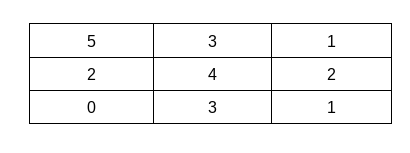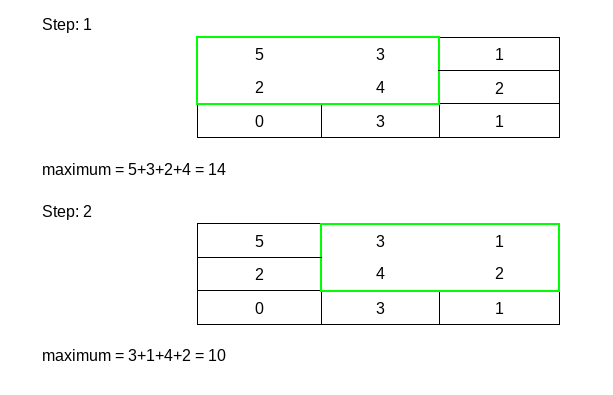The matrices in the highlighted box are the submatrices we counted. Maximum sum is 14 and the submatrix is, [ {5,3}, {2,4} ].
The same process can be used to find the maximum/minimum value in a matrix, calculate an average, and find a specific pair of submatrices.

For finding the minimum elements from the matrix, we will compare each element with the previous one to find the minimum among them.
The first element will not change and window size = 2.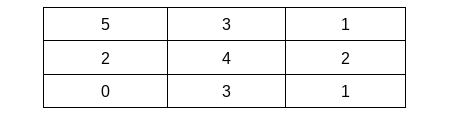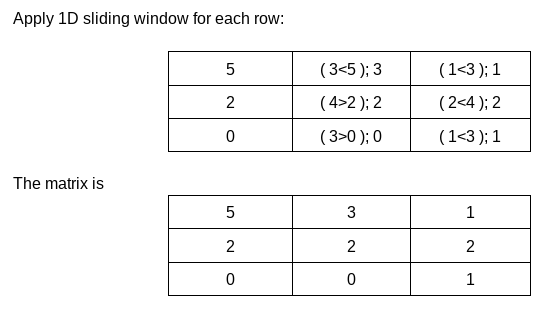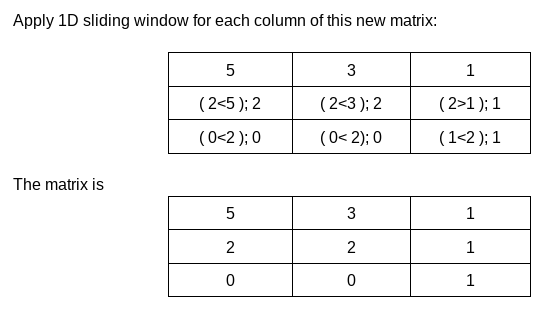This is the minimum matrix.

Another approach to applying a sliding window on a 2D array is to convert the 2D array into a 1D array. Then we will only need to apply the 1D sliding window. The process will be easier.

# Time and Space Complexity of 2D Sliding Window

Time complexity indicates the time an algorithm takes to complete a task. Similarly, space complexity refers to the memory used by the algorithm for completing the task.
A program runs faster if these two can be reduced. Time and space complexities will be low depending on the simplicity of the process.

For traversing through the 2D array, as every element of every cell will be accessed,
Time complexity = O(number of rows x number of column)

As this will take some temporary space, they will not be calculated in the space complexity. They are auxiliary space. So the space complexity will remain constant.
Space complexity = O(1)

With this article at OpenGenus, you must have the complete idea of 2D Sliding Window.#### Muhsina Munfa

Muhsina Munfa is a graduate with a BSc degree in the Department of CSE from Metropolitan University Sylhet.